Goseeko blog# What is Frequency Modulation?

Frequency Modulation is the technique in which the frequency of a carrier signal is varied according to the message signal.  Basically, when the frequency or phase of a carrier signal is varied according to the message signal it is called Angle Modulation.

The equation for angle modulated wave is given as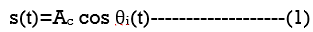Where:

Ac : It is the amplitude of modulated wave

θi(t) : Angle of modulated wave

## Frequency Modulation

In amplitude modulation, the amplitude of the carrier signal varies, whereas in Frequency Modulation (FM), the frequency of the carrier signal is varied according to the instantaneous amplitude of the message signal. Hence, in frequency modulation, only frequency is varied keeping the amplitude and phase of the carrier signal constant.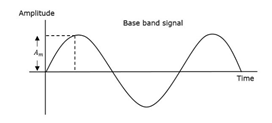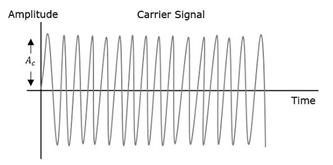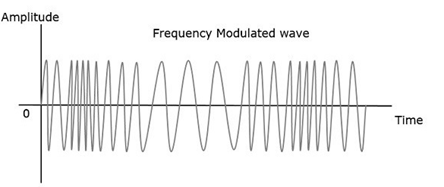• The rate at which the carrier frequency will increase or decrease is controlled by the frequency of the message signal (fi ). Unlike amplitude modulation where fi < fC and the amplitude is constant for all time.
• The change in frequency of the carrier will also reach its maximum deviation which is above the normal value when the message signal’s voltage reaches its maximum value.
• The change in frequency of the carrier will also reach its minimum deviation which is below  the normal value when the message signal’s voltage reaches its minimum value.
• There will be no deviation in the carrier when the information signal is zero.
• There will not be any deviation of the carrier when there is no information which means the information signal is zero. The voltage frequency deviation is set when maximum change occurs in the carrier from the base value.

### Equation of Frequency Modulation

The equation for instantaneous frequency fi in FM modulation will then become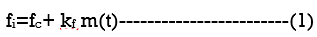Where:

fc is the carrier frequency

kf is the frequency sensitivity

m(t) is the message signal

Then relation between the two is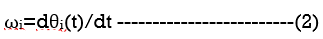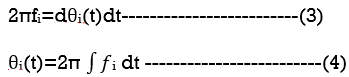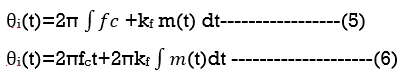Then the equation of FM wave is written above. If the modulating signal is m(t)=Am cos(2πfmt) then the equation of FM wave will be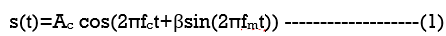Where: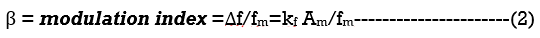The difference between FM modulated frequency which is the instantaneous frequency and normal carrier frequency is termed as Frequency Deviation. It is denoted by Δf which is equal to

Δf =  kf  Am.

Based on the values of modulation index β there are two types of frequency modulation techniques Narrowband FM and Wideband FM

Interested in learning about similar topics? Here are a few hand-picked blogs for you!